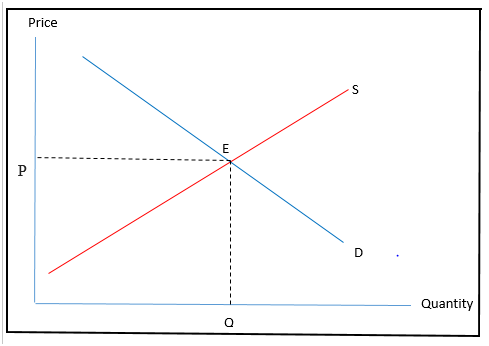# How to Find Equilibrium Price and Quantity

Equilibrium is the situation where we can see the equality of market demand quantity and supply quantity. In other words, it is a situation where an economy shows the equality of two opposite market forces. Economic equilibrium is a situation of the balance of economic forces and in this article, we’ll talk about the equilibrium Price and Quantity. It further illustrates the circumstance where the point supply equals to demand of a product with the behavior of equilibrium price and quantity determined at the point in which supply and demand curves intersect.  We can see this situation in the standard textbook model of Perfect competition in economy and equilibrium can be described as below.

## How to Find Equilibrium Price and Quantity

Market equilibrium can be calculated in various ways. In this article, we’ll look at the following methods.

1. Demand and Supply schedule
2. Demand and Supply curves
3. Demand and supply formulas

### Demand and Supply Schedule

Demand and Supply schedule shows the sample of market demand and supply as well as the price level relevant to different stages.

 Price Level Quantity of Demand (QD) Quantity of Supply (QS) 0 300 0 5 250 50 10 200 100 15 150 150 20 100 200 25 50 250 30 0 30

Where, P = Price, QD = Quantity demanded and QS = Quantity supplied,

According to the figures in the given table, Market Equilibrium quantity is 150 and the Market equilibrium price is 15. It is the point where QD = QS, of the given figures.

### Demand and Supply Curves

This is the way how economist use demand and supply curves to prove the market equilibrium. This is a graphical representation of the market behavior and clearly shows the intersection point in the graph itself.

Using the previous demand and supply schedule we can create market equilibrium as below.You can see the competitive equilibrium in above curve as 150 quantities and the price of LKR15.00 in this curve blue color line shows market demand and the orange color line shows market supply.

• P – price
• Q – quantity demanded and supplied
• S – supply curve
• D – demand curve
• QE – equilibrium price

### Demand and Supply Formulas

With the same example let’s see how to calculate market equilibrium as below.

QD = Quantity demanded

QS = Quantity supplied

P = Price

Condition: At the equilibrium point quantity demanded equals to the quantity supplied.

QD = QS

By substituting demand and supply formula to the given example equilibrium quantity and price can be calculated.

Demand formula QD = a- bp

Supply formula QS = a + bp

a is the intercept of the demand and supply curves. In other words, it is the demand and supply quantities at price zero. b is the slope of two curves. This can be calculated by ΔQ / ΔP. Therefore, demand and supply equations can be formulated as follows.

QD = 300 – 10P,

QS = 0 + 10P

### How to Find Equilibrium Price

QD                  =       QS

300-10p         =   0 +10P

300 / 20         =   20P / 20

P                    =  15

By substituting P and Q values to both demand and supply equations, equilibrium price and quantity can be found as follows.

QD = 300-10P                                                 QS = 0+10P

QD = 300-10x 15                                             QS = 0+10×15

QD = 150                                                          QS = 150

## What are the Special Features of Equilibrium in a Competitive Market

The equilibrium shows following special features in a competitive market. These features can be used to identify and measure the market equilibrium.

1. Demand quantity is always equal to Supply quantity.
2. Demand price of the competitive economy is always equal to the price of supply.
3. There’s no high demand or high supply.
4. All the external forces which can influence the price and quantity is always absence in this kind of condition.
5. The consumer planned quantity is equal to the planned prices of supply.

### Conclusion

Equilibrium is always related to demand quantity and supply quantity. Market equilibrium can be found using supply and demand schedule, demand and supply curves and formula of demand and supply. The condition of market equilibrium shows the absence of external forces which can influence the price as well as quantity.